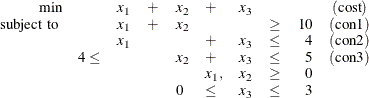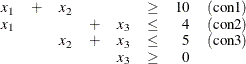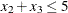### Example 6.4 Finding an Irreducible Infeasible Set

This example demonstrates the use of the IIS= option to locate an irreducible infeasible set. Suppose you want to solve a linear program that has the following simple formulation:It is easy to verify that the following three constraints (or rows) and one variable (or column) bound form an IIS for this problem:You can formulate the problem and call the LP solver by using the following statements:

proc optmodel presolver=none;
/* declare variables */
var x{1..3} >=0;

/* upper bound on variable x */
x.ub = 3;

/* objective function */
min obj = x + x + x;

/* constraints */
con c1: x + x >= 10;
con c2: x + x <= 4;
con c3: 4 <= x + x <= 5;

solve with lp / iis = on;

print x.status;
print c1.status c2.status c3.status;


The notes printed in the log appear in Output 6.4.1.

Output 6.4.1: Finding an IIS: Log

 NOTE: Problem generation will use 4 threads. NOTE: The problem has 3 variables (0 free, 0 fixed). NOTE: The problem has 3 linear constraints (1 LE, 0 EQ, 1 GE, 1 range). NOTE: The problem has 6 linear constraint coefficients. NOTE: The problem has 0 nonlinear constraints (0 LE, 0 EQ, 0 GE, 0 range). NOTE: The IIS option is enabled. Objective Phase Iteration        Value         Time P 1          1    1.400000E+01         0 P 1          4    1.000000E+00         0 NOTE: The IIS option found the problem to be infeasible. NOTE: Applying the IIS sensitivity filter. NOTE: The sensitivity filter removed 1 constraints and 3 variable bounds. NOTE: Applying the IIS deletion filter. NOTE: Processing constraints. Processed     Removed      Time 0           0         0 1           0         0 2           0         0 3           0         0 NOTE: Processing variable bounds. Processed     Removed      Time 0           0         0 1           0         0 2           0         0 3           0         0 NOTE: The deletion filter removed 0 constraints and 0 variable bounds. NOTE: The IIS option found the problem to be infeasible. NOTE: The IIS option found an irreducible infeasible set with 1 variables and 3 constraints. NOTE: The IIS solve time is 0.02 seconds.

The output of the PRINT statements appears in Output 6.4.2. The value of the .status suffix for the variables x and x is I, which indicates an infeasible problem. The value I is not one of those assigned by the IIS= option to members of the IIS, however, so the variable bounds for x and x are not in the IIS.

Output 6.4.2: Solution Summary, Variable Status, and Constraint Status

The OPTMODEL Procedure

Solution Summary
Solver LP
Algorithm Primal Simplex
Objective Function obj
Solution Status Infeasible

Iterations 14
Presolve Time 0.00
Solution Time 0.05

 x.STATUS
1
2
3 I_L

c1.STATUS c2.STATUS c3.STATUS
I_L I_U I_U

The value of c3.status is I_U, which indicates thatis an element of the IIS. The original constraint is c3, a range constraint with a lower bound of 4. If you choose to remove the constraint, you can change the value of c3.ub to the largest positive number representable in your operating environment. You can specify this number by using the MIN aggregation expression in the OPTMODEL procedure. See MIN Aggregation Expression for details.

The modified LP problem is specified and solved by adding the following lines to the original PROC OPTMODEL call.

   /* relax upper bound on constraint c3 */
c3.ub = min{{}}0;

solve with lp / iis = on;

/* print solution */
print x;



Because one element of the IIS has been removed, the modified LP problem should no longer contain the infeasible set. Due to the size of this problem, there should be no additional irreducible infeasible sets.

The notes shown in Output 6.4.3 are printed to the log.

Output 6.4.3: Infeasibility Removed: Log

 NOTE: Problem generation will use 4 threads. NOTE: The problem has 3 variables (0 free, 0 fixed). NOTE: The problem has 3 linear constraints (1 LE, 0 EQ, 2 GE, 0 range). NOTE: The problem has 6 linear constraint coefficients. NOTE: The problem has 0 nonlinear constraints (0 LE, 0 EQ, 0 GE, 0 range). NOTE: The IIS option is enabled. Objective Phase Iteration        Value         Time P 1          1    1.400000E+01         0 P 1          3    0.000000E+00         0 NOTE: The IIS option found the problem to be feasible. NOTE: The IIS solve time is 0.05 seconds.

The solution summary and primal solution are displayed in Output 6.4.4.

Output 6.4.4: Infeasibility Removed: Solution

The OPTMODEL Procedure

Solution Summary
Solver LP
Algorithm Primal Simplex
Objective Function obj
Solution Status Feasible

Iterations 3
Presolve Time 0.00
Solution Time 0.03

 x
1 0
2 0
3 0